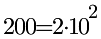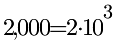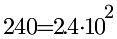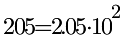# Convert Standard Numbers to Scientific Notation - Set 1

Explanation: The numbers 50 and 10,000 are called, "standard numbers".  When numbers are written in "scientific notation" the first factor is a number is between 1 and 10 and the second factor is a power of 10.   The exponent for the 10 matches the number of spaces the decimal point needs to move. Observe these conversions of "standard numbers" to "scientific notation".

Examples:Directions: For this problem set you will need to analyze the "standard number" and choose the equivalent "scientific notation".  Strive to be able to make the decisions with ease and then strive to increase your speed.  Good luck and enjoy the challenge!

...